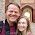## Monday, December 10, 2012

### Checking for differences in estimates by group

Stata do file

* Imagine that you have two groups in your sample and you are wondering if both groups respond the explanatory variables in the same manner.

clear
set obs 1000

gen group=rbinomial(1,.5)

gen x = rnormal()

gen y = 2 + 3*x + rnormal()*2 if group==0
replace y = 1 + 0*x + rnormal()*2 if group==1

* There is several ways to test if the two sets of estimators are the same.

* One method is using interaction dummies.

gen x_group = x*group

* The technique is to include an interaction term in the model for each coefficient that you would like to check if it is equal.

* Group counts as an interaction term between the constant and the group indicator.
* x_group is the interaction between the x variable and the group variable.

reg y group x_group x

* In order to interpret the coefficients in the above regression.

* First set group equal to zero.

* In that case all that is in the regression is: y = 1*b0 + x*b1 which is the estimator for the group 0.

* We can see the constant estimate is close to 2 and the coefficient on x is close to 3 which are our values.

* Now the tricky thing is to interpret the remaining coefficients.

* Because the group coefficient is effectively interacted with the constant then in order to estimate the constant in the group=1 set just add _b[group- to _b[cons].

di "Group 2 constant estimate is " _b[group]+ _b[_cons] " which we can see is close to 1"

* We do a similar procedure with the slope.

di "Group 2 slope estimate is " _b[x]+ _b[x_group] " which we can see is close to 0"

* Thus we can easily test the hypothesis that both are the same manner.

test x_group=group=0

* If both groups were the same then they would have the same mean (same constants) and respond the same the the treatment x.

* An alternative way of testing each coefficient individually is:

reg y x if group == 0

local b_cong0 = _b[_cons]
local b_xg0 = _b[x]
local se_cong0 = _se[_cons]
local se_xg0 = _se[x]

reg y x if group == 1

* The t-test that they are the same is b[group=0]-b[group=1]=0
* var(b[group=0]-b[group=1])= var(b[group=0]) + var(b[group=1])

local joint_var_cons = se_cong0'^2 + _se[_cons]^2

* And the t test is (b[group=0]-b[group=1])/(var(b[group=0]) + var(b[group=1]))^.5

di "t_constants = " (b_cong0'- _b[_cons] )/(joint_var_cons')^.5

local joint_var_x= se_xg0'^2 + _se[x]^2

di "t_constants = " (b_xg0'-_b[x])/joint_var_x'^.5

* This formation assumes that the estimates vary independently.

* If done properly the t-stats are almost indentical between formulations.

* By the way, please fill out my survey posted on the last post.

1.1.2.